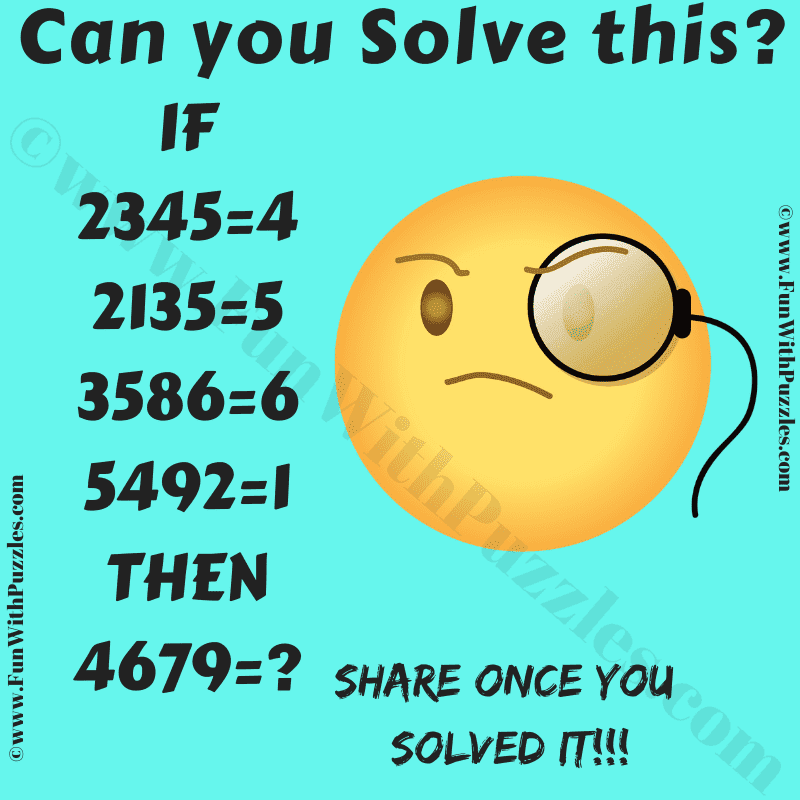It is a logical maths puzzle for school students. Solving this puzzle will help you to improve your IQ. Your logical skills will get improved if you solve such maths logic puzzles on regular basis. Anyway in this puzzle, there are logical equations. Your challenge in these equations is to find the hidden logic and then solve the last logical equation. Can you crack the hidden logic in this equation puzzle and find the value of the missing number in the last equation?Can you solve this Logical Puzzle?

The answer to this "Maths Puzzle", can be viewed by clicking on the answer button.